Next: Principal Components Analysis Up: Temporal Modeling Previous: Temporal Modeling

# Time Series

A detailed account of the Santa Fe competition is presented in . The document covers many of the issues involved in time series modeling and prediction that are relevant to the objectives posed above. In this collected work , multiple signal types are considered with rather general tools as well as domain-specific approaches. An important insight results: the representation of the time-series signal critically depends on the machine learning engines that it will feed into. In that sense, the temporal representation of data can be treated as a pre-processing engine that feeds a specific learning system. The learning stage obtains data and forms a model that is optimized to predict future time series values from present ones. This task falls into the general category of regression which learns a mapping between inputs and outputs, effectively approximating a function and computing a new output given a new input. In the case of time series, the input is a past sequence and the output is the subsequent sequence (i.e. the forecast or prediction).

While regression has traditionally been associated with linear models and ARMA (autoregressive moving average) processing, the Santa Fe results note the weakness of linear models and the over-simplifying assumptions they imply. These classical techniques are contrasted with the far more succesful neural and connectionist approaches. This more recent modeling paradigm uses fundamentally non-linear techniques for signal prediction. This non-linear nature is necessary since the data employed in the competition ranged over complex non-linear phenomena such as physiological signals and Bach's unfinished compositions. Similarly, we argue that we have no good reason to assume that the perceptually recovered gestures and behaviours in the ARL system should be linear as well. At this point we examine the neural or connectionist approaches for time series forecasting and for representation. These ideas will be used to motivate the design of the ARL learning system and the representations it will employ. We focus on the connectionist approach due to its explicit non-linear optimization of prediction accuracy and its superior performance in the Santa Fe competition against systems like hidden Markov models, dynamic models, and so on.

In his implementation of a Time Delay Neural Network (TDNN), Wan  contrasts this time series based connectionist architecture with the standard static multi-layer neural network. In theory, the nonlinear autoregression being computed is a mapping between an output vector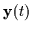and T previous instances of the vector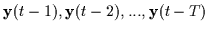as in Equation 4.1. The latest observation here is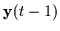and the oldest is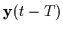. The neural network has to find an approximation to the function g() which is often denoted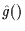. In our case,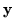is a 30 dimensional vector containing the perceptual parameters recovered from the two human teachers in the training mode. Each user generates 3 Gaussian blobs (head and hands) and these 30 parameters are merely concatenated into a single vector.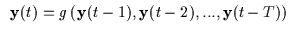(4.1)

Thus, the function g() produces an outputwhich is 30 dimensional for the head and hand tracking case but requires Tprevious vector observations of. Thus, the input space of g() is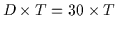. If implemented as a regular multi-layer neural network (static) as in Figure 4.1, there is a large increase in complexity with increasing past observation vectors T that can be stored simultaneously.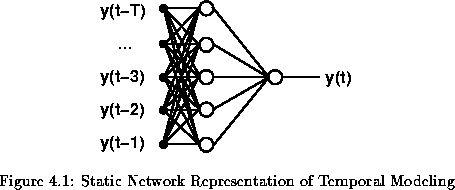The T value represents the number of observation vectors in the system's memory and these will determine how much past data it may use to make forecasts about the immediate future. For head and hand tracking data (which generates vectors at roughly 15Hz), values of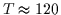are required to form a short term memory of a few seconds (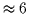seconds). Thus, the input domain of the neural function g() would grow to over 3600 dimensions.

Wan discusses an algorithm which treats the time series using finite impulse response (FIR) neurons and symmetries on a static network topology to reduce the dimensionality problem and take advantage of some symmetries and redundancies. We shall now divert from his methodology and address the large dimensionality of the input space using an alternative approach.Next: Principal Components Analysis Up: Temporal Modeling Previous: Temporal Modeling
Tony Jebara
1999-09-15